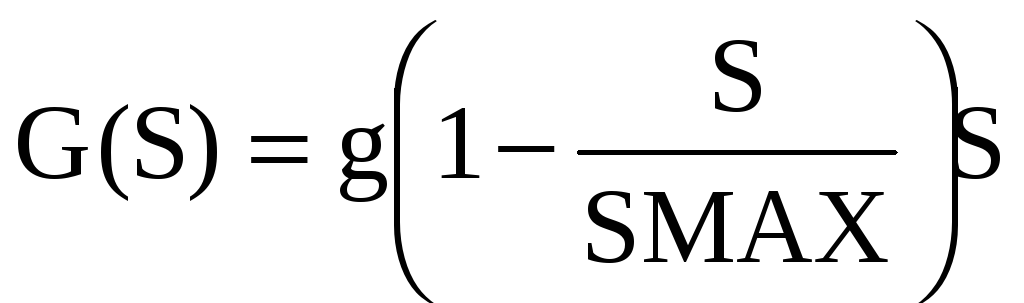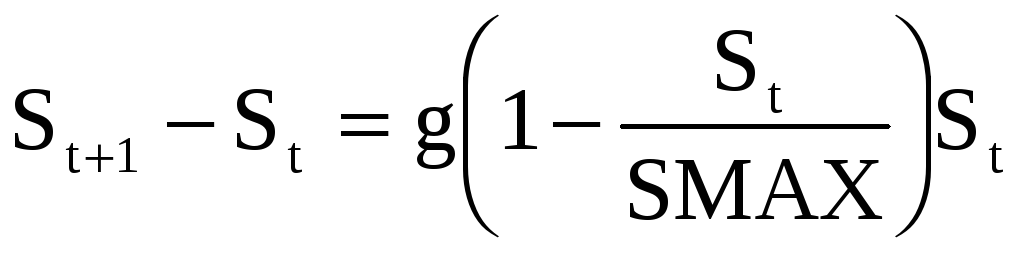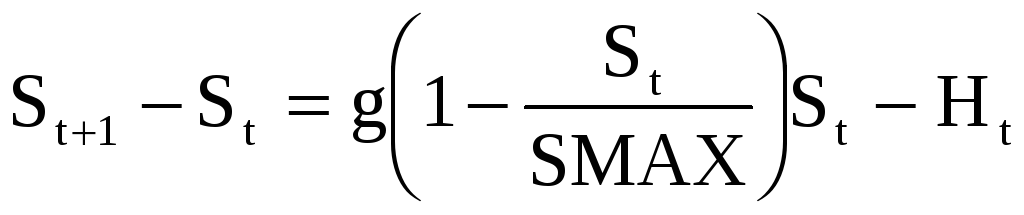# Chapter 9: comparative statics of fishery economics

 Page 1/7 Date 04.06.2018 Size 180.5 Kb.
File: exploit6.doc

Date: 7 July 2000

CHAPTER 9: COMPARATIVE STATICS OF FISHERY ECONOMICS
We begin by laying out the equations that are used in the fishery models covered in the textbook and in these additional materials. The analysis in the chapter text uses continuous time notation. This convention is largely followed in this Word file. However, when we use Excel for dynamic simulation (as in exploit5.xls) we necessarily adopt discrete time, as spreadsheets are set up to do calculation recursively in discrete time intervals. To help the reader, we give the reader the model specifications in both forms (although our derivations are done here only in continuous time).
THE FISHERY MODELS: GENERAL FUNCTION SPECIFICATION
 Continuous Time Model Discrete Time Model Pure biological growth (1) dS/dt = G(S) St+1-St = G(St) Fishery production function (2) H = H(E, S) Ht = H(Et, St) Net growth of fish stock (3) dS/dt = G(S) - H St+1-St = G(St) - Ht Fishery revenue (4) B = PH, P constant Bt = PHt, P constant Fishery costs (5) C = C(E) Ct = C(Et) Fishery profit (6) NB = B - C NBt = Bt - Ct FISHING EFFORT DYNAMICS Open access entry rule (7) dE/dt = dNB Et+1-Et =dNBt Private property entry rule (8) dE/dt = d(dNB/dE)*E Et+1-Et =d(dNBt/dEt)*E STEADY STATE CONDITIONS Biological equilibrium (9) G = H Gt = Ht Economic equilibrium (10) E(t) = E* Et+1 = Et = E*

FISHERY MODEL: WITH ASSUMED FUNCTIONAL FORMS
 Continuous Time Model Discrete Time Model Pure biological growth (1)Fishery production function (2) H = eES Ht = eEtSt Net growth of fish stock (3)- HFishery revenue (4) B = PH, P constant Bt = PHt, P constant Fishery costs (5) C =wE Ct =wEt Fishery profit (6) NB = B - C = PeES -wE NBt = Bt - Ct = PeEtSt -wEt FISHING EFFORT DYNAMICS